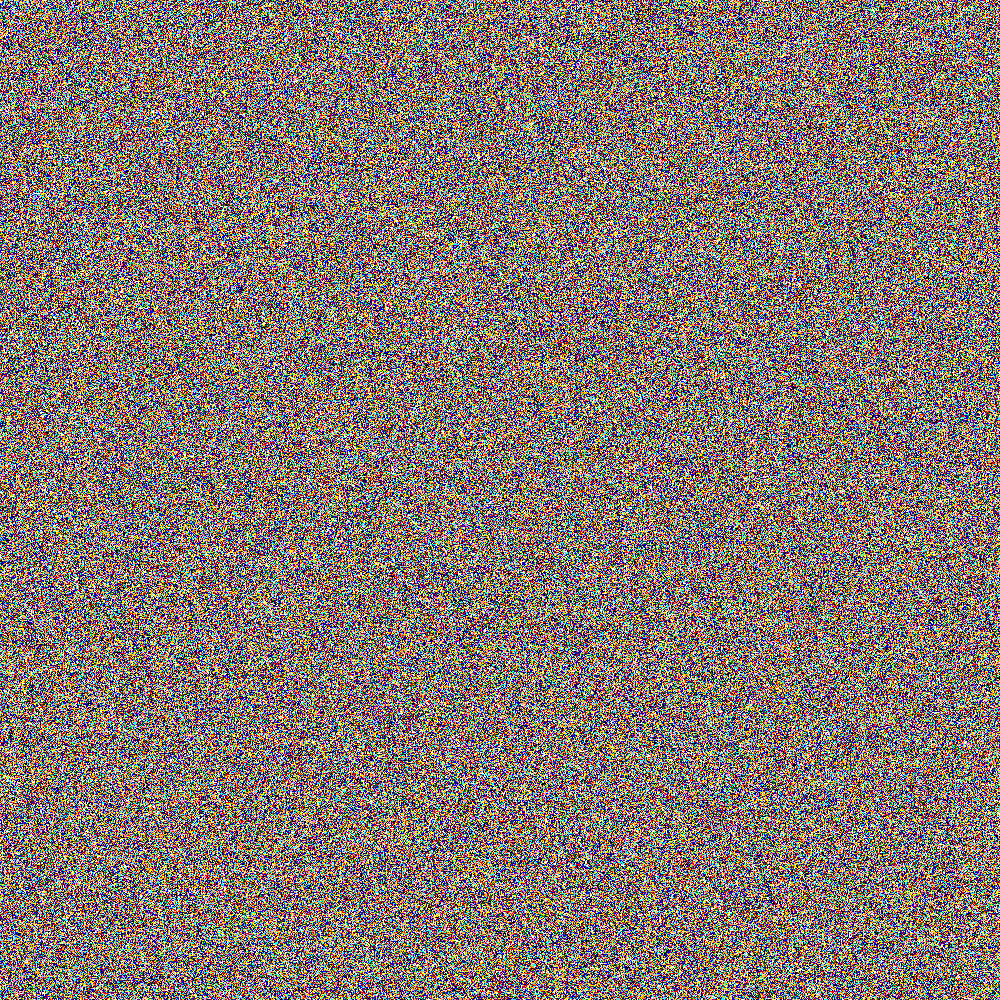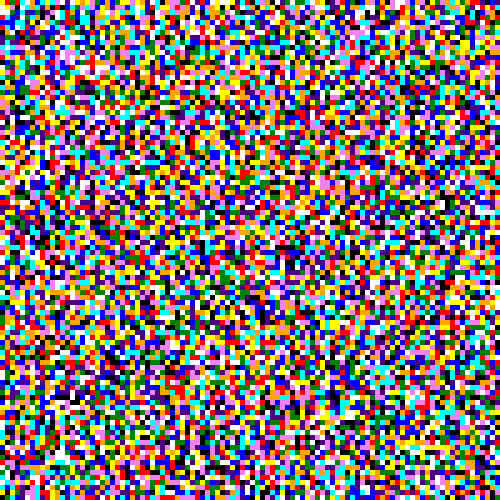# Pi Visualization

example p5.js javascript arrays

This sketch assigns a different color to every digit 0-9, and then draws a single pixel for each of the first million digits of pi.

Edit this code in the p5.js editor.

``````let piString;

const columns = 1000;
const rows = 1000;
let row = 0;

let colors = [];

}

piString = piLines;
}

function setup() {
createCanvas(1000, 1000);
noSmooth();
noStroke();
background(32);

colors = [
"black", //0
"red", //1
"orange", //2
"yellow", //3
"green", //4
"cyan", // 5
"blue", //6
"indigo", //7
"violet", //8
"white", //9
];
}

// restart the animation with random colors
function keyPressed() {
colors = [];
for (let i = 0; i < 10; i++) {
colors.push(color(random(255), random(255), random(255)));
}
background(0);
row = 0;
loop();
}

function draw() {
if (!piString) {
return;
}

// draw a whole row of digits
for (let column = 0; column < columns; column++) {
const piDigitIndex = columns * row + column;
const piDigit = int(piString.substring(piDigitIndex, piDigitIndex + 1));
const digitColor = colors[piDigit];

fill(digitColor);
rect(column, row, 1, 1);
}

// move to the next row, or stop the animation
row++;
if (row >= rows) {
console.log("Done!");
noLoop();
}
}
``````

Edit this code in the p5.js editor.If this looks like randomness, that’s because it is! The digits in pi are random, or at least mostly random. Don’t ask me, I just think it looks neat.

Zoomed in:Other colors:# Remix Ideas

• Visualize a different number, like Euler’s number or the golden ratio
• Instead of taking a single digit at a time, what would it look like to take 2 or 3 digits at a time?
• What would it look like to play connect-the-dots with all of the threes?